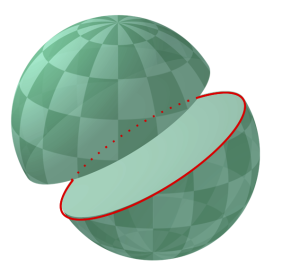# GPU Pro Tip: Fast Great-Circle Distance Calculation in CUDA C++This post demonstrates the practical utility of CUDA’s `sinpi()` and `cospi()` functions in the context of distance calculations on earth. With the advent of location-aware and geospatial applications and geographical information systems (GIS), these distance computations have become commonplace.A great circle divides a sphere into two hemispheres. Source: Wikimedia Commons

Wikipedia defines a great circle as

A great circle, also known as an orthodrome or Riemannian circle, of a sphere is the intersection of the sphere and a plane which passes through the center point of the sphere.

For almost any pair of points on the surface of a sphere, the shortest (surface) distance between these points is the path along the great circle between them. If you have ever flown from Europe to the west coast of North America and wondered why you passed over Greenland, your flight most likely followed a great circle path in order to conserve fuel.

Following is the code for a C function, `haversine()`, which computes the great-circle distance of two points on earth (or another sphere), using the Haversine formula. People differ on their philosophy as to the “correct” radius when assuming a spherical earth (given that earth is not a sphere; Wikipedia provides some guidance on this matter). Therefore, the earth’s radius is an input to the function, which also allows trivial switching between kilometers or miles as units. The accuracy of the formula when computed in single-precision should generally be fully adequate for distance computations within a continent.

```/* This function computes the great-circle distance of two points on earth
using the Haversine formula, assuming spherical shape of the planet. A
well-known numerical issue with the formula is reduced accuracy in the
case of near antipodal points.

lat1, lon1: latitude and longitude of first point, in degrees [-90, +90]
lat2, lon2: latitude and longitude of second point, in degrees [-180, +180]
radius: radius of the earth in user-defined units, e.g. 6378.2 km or
3963.2 miles

returns: distance of the two points, in the same units as radius

Reference: http://en.wikipedia.org/wiki/Great-circle_distance
*/
__device__ float haversine (float lat1, float lon1,
float lat2, float lon2, float radius)
{
float dlat, dlon, c1, c2, d1, d2, a, c, t;

c1 = cospif (lat1 / 180.0f);
c2 = cospif (lat2 / 180.0f);
dlat = lat2 - lat1;
dlon = lon2 - lon1;
d1 = sinpif (dlat / 360.0f);
d2 = sinpif (dlon / 360.0f);
t = d2 * d2 * c1 * c2;
a = d1 * d1 + t;
c = 2.0f * asinf (fminf (1.0f, sqrtf (a)));
return radius * c;
}```

Thanks to Norbert Juffa for contributing this code.